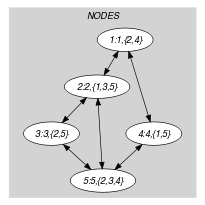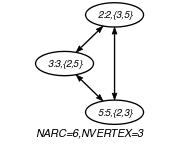## 5.71. clique

Origin
Constraint

$\mathrm{𝚌𝚕𝚒𝚚𝚞𝚎}\left(\mathrm{𝚂𝙸𝚉𝙴}_\mathrm{𝙲𝙻𝙸𝚀𝚄𝙴},\mathrm{𝙽𝙾𝙳𝙴𝚂}\right)$

Arguments
 $\mathrm{𝚂𝙸𝚉𝙴}_\mathrm{𝙲𝙻𝙸𝚀𝚄𝙴}$ $\mathrm{𝚍𝚟𝚊𝚛}$ $\mathrm{𝙽𝙾𝙳𝙴𝚂}$ $\mathrm{𝚌𝚘𝚕𝚕𝚎𝚌𝚝𝚒𝚘𝚗}\left(\mathrm{𝚒𝚗𝚍𝚎𝚡}-\mathrm{𝚒𝚗𝚝},\mathrm{𝚜𝚞𝚌𝚌}-\mathrm{𝚜𝚟𝚊𝚛}\right)$
Restrictions
 $\mathrm{𝚂𝙸𝚉𝙴}_\mathrm{𝙲𝙻𝙸𝚀𝚄𝙴}\ge 0$ $\mathrm{𝚂𝙸𝚉𝙴}_\mathrm{𝙲𝙻𝙸𝚀𝚄𝙴}\le |\mathrm{𝙽𝙾𝙳𝙴𝚂}|$ $\mathrm{𝚛𝚎𝚚𝚞𝚒𝚛𝚎𝚍}$$\left(\mathrm{𝙽𝙾𝙳𝙴𝚂},\left[\mathrm{𝚒𝚗𝚍𝚎𝚡},\mathrm{𝚜𝚞𝚌𝚌}\right]\right)$ $\mathrm{𝙽𝙾𝙳𝙴𝚂}.\mathrm{𝚒𝚗𝚍𝚎𝚡}\ge 1$ $\mathrm{𝙽𝙾𝙳𝙴𝚂}.\mathrm{𝚒𝚗𝚍𝚎𝚡}\le |\mathrm{𝙽𝙾𝙳𝙴𝚂}|$ $\mathrm{𝚍𝚒𝚜𝚝𝚒𝚗𝚌𝚝}$$\left(\mathrm{𝙽𝙾𝙳𝙴𝚂},\mathrm{𝚒𝚗𝚍𝚎𝚡}\right)$ $\mathrm{𝙽𝙾𝙳𝙴𝚂}.\mathrm{𝚜𝚞𝚌𝚌}\ge 1$ $\mathrm{𝙽𝙾𝙳𝙴𝚂}.\mathrm{𝚜𝚞𝚌𝚌}\le |\mathrm{𝙽𝙾𝙳𝙴𝚂}|$
Purpose

Consider a digraph $G$ described by the $\mathrm{𝙽𝙾𝙳𝙴𝚂}$ collection: to the ${i}^{th}$ item of the $\mathrm{𝙽𝙾𝙳𝙴𝚂}$ collection corresponds the ${i}^{th}$ vertex of $G$; To each value $j$ of the ${i}^{th}$ $\mathrm{𝚜𝚞𝚌𝚌}$ variable corresponds an arc from the ${i}^{th}$ vertex to the ${j}^{th}$ vertex. Select a subset $𝒮$ of the vertices of $G$ that forms a clique of size $\mathrm{𝚂𝙸𝚉𝙴}_\mathrm{𝙲𝙻𝙸𝚀𝚄𝙴}$ (i.e., there is an arc between each pair of distinct vertices of $𝒮$).

Example
$\left(\begin{array}{c}3,〈\begin{array}{cc}\mathrm{𝚒𝚗𝚍𝚎𝚡}-1\hfill & \mathrm{𝚜𝚞𝚌𝚌}-\varnothing ,\hfill \\ \mathrm{𝚒𝚗𝚍𝚎𝚡}-2\hfill & \mathrm{𝚜𝚞𝚌𝚌}-\left\{3,5\right\},\hfill \\ \mathrm{𝚒𝚗𝚍𝚎𝚡}-3\hfill & \mathrm{𝚜𝚞𝚌𝚌}-\left\{2,5\right\},\hfill \\ \mathrm{𝚒𝚗𝚍𝚎𝚡}-4\hfill & \mathrm{𝚜𝚞𝚌𝚌}-\varnothing ,\hfill \\ \mathrm{𝚒𝚗𝚍𝚎𝚡}-5\hfill & \mathrm{𝚜𝚞𝚌𝚌}-\left\{2,3\right\}\hfill \end{array}〉\hfill \end{array}\right)$

The $\mathrm{𝚌𝚕𝚒𝚚𝚞𝚎}$ constraint holds since the $\mathrm{𝙽𝙾𝙳𝙴𝚂}$ collection depicts a clique involving 3 vertices (namely vertices 2, 3 and 5) and since its first argument $\mathrm{𝚂𝙸𝚉𝙴}_\mathrm{𝙲𝙻𝙸𝚀𝚄𝙴}$ is set to the number of vertices of this clique.

Typical
 $\mathrm{𝚂𝙸𝚉𝙴}_\mathrm{𝙲𝙻𝙸𝚀𝚄𝙴}\ge 2$ $\mathrm{𝚂𝙸𝚉𝙴}_\mathrm{𝙲𝙻𝙸𝚀𝚄𝙴}<|\mathrm{𝙽𝙾𝙳𝙴𝚂}|$ $|\mathrm{𝙽𝙾𝙳𝙴𝚂}|>2$
Symmetry

Items of $\mathrm{𝙽𝙾𝙳𝙴𝚂}$ are permutable.

Arg. properties

Functional dependency: $\mathrm{𝚂𝙸𝚉𝙴}_\mathrm{𝙲𝙻𝙸𝚀𝚄𝙴}$ determined by $\mathrm{𝙽𝙾𝙳𝙴𝚂}$.

Algorithm

[Fahle02][Regin03CPAIOR], [Regin03]. The algorithm for finding maximum cliques in an undirected graph of C. Bron and J. Kerbosch [BronKerbosch73] was adapted by J.-C. Régin to the context of constraint programming in his papers.

Keywords
Arc input(s)

$\mathrm{𝙽𝙾𝙳𝙴𝚂}$

Arc generator
$\mathrm{𝐶𝐿𝐼𝑄𝑈𝐸}$$\left(\ne \right)↦\mathrm{𝚌𝚘𝚕𝚕𝚎𝚌𝚝𝚒𝚘𝚗}\left(\mathrm{𝚗𝚘𝚍𝚎𝚜}\mathtt{1},\mathrm{𝚗𝚘𝚍𝚎𝚜}\mathtt{2}\right)$

Arc arity
Arc constraint(s)
$\mathrm{𝚒𝚗}_\mathrm{𝚜𝚎𝚝}$$\left(\mathrm{𝚗𝚘𝚍𝚎𝚜}\mathtt{2}.\mathrm{𝚒𝚗𝚍𝚎𝚡},\mathrm{𝚗𝚘𝚍𝚎𝚜}\mathtt{1}.\mathrm{𝚜𝚞𝚌𝚌}\right)$
Graph property(ies)
 $•$$\mathrm{𝐍𝐀𝐑𝐂}$$=\mathrm{𝚂𝙸𝚉𝙴}_\mathrm{𝙲𝙻𝙸𝚀𝚄𝙴}*\mathrm{𝚂𝙸𝚉𝙴}_\mathrm{𝙲𝙻𝙸𝚀𝚄𝙴}-\mathrm{𝚂𝙸𝚉𝙴}_\mathrm{𝙲𝙻𝙸𝚀𝚄𝙴}$ $•$$\mathrm{𝐍𝐕𝐄𝐑𝐓𝐄𝐗}$$=\mathrm{𝚂𝙸𝚉𝙴}_\mathrm{𝙲𝙻𝙸𝚀𝚄𝙴}$

Graph class
$\mathrm{𝚂𝚈𝙼𝙼𝙴𝚃𝚁𝙸𝙲}$

Graph model

Note the use of set variables for modelling the fact that the vertices of the final graph have more than one successor: The successor variable associated with each vertex contains the successors of the corresponding vertex.

Part (A) of Figure 5.71.1 shows the initial graph from which we start. It is derived from the set associated with each vertex. Each set describes the potential values of the $\mathrm{𝚜𝚞𝚌𝚌}$ attribute of a given vertex. Part (B) of Figure 5.71.1 gives the final graph associated with the Example slot. Since we both use the $\mathrm{𝐍𝐀𝐑𝐂}$ and $\mathrm{𝐍𝐕𝐄𝐑𝐓𝐄𝐗}$ graph properties, the arcs and the vertices of the final graph are stressed in bold. The final graph corresponds to a clique containing three vertices.

##### Figure 5.71.1. Initial and final graph of the $\mathrm{𝚌𝚕𝚒𝚚𝚞𝚎}$ set constraint(a) (b)